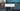# Min Priority Queue Implementation with Heap Data structure

July 10, 2019Min Priority Queue is a data structure which manage a list of keys(values). And, gives priority to element with minimum value.

It supports following operations:

1. getMin() - Gives the minimum priority element. Do not delete it.
2. extractMin() - Gives the minimum priority element, and delete it.
3. insert(element) - Insert an element into Priority queue
4. decrement(index, newValue) - Decrement an element from priority queue

(Heap data structure)[/coding-interview/what-is-heap-data-structure/] can be used for its implementation.

## Why not other data structure (other than Heap)?

Lets see each one of them:

• Link list Runtime complexity is O(n^2) in putting minimum element at top each time, a new element has come. Or, priority change for an element.
• Binary Search Tree Insertion is easy, getting minimum is an easy operation. It consumes extra space for keeping pointers for each node. In case of insertion and changing priority, tree needs to be re-balanced again, which is more complex than maintaining min heap property in heap data structure. Heap uses arrays, so accessing an element, caching an element are always faster operation.

## Implementation

``````public class MinPriorityQueue {
private int[] heap;
private int maxSize;
private int heapSize;

public MinPriorityQueue(int maxSize) {
this.maxSize = maxSize;
this.heap = new int[maxSize];
this.heapSize = 0;
}

@Override
public String toString() {
return ArrayUtils.toString(this.heap, this.heapSize);
}

private int getLeftChild(int index) { return 2*index + 1;}
private int getRightChild(int index) { return 2*index + 2;}
private int getParent(int index) {
if (index == 0) {
return -1;
}
return (index-1)/2;
}

private void minHeapify(int index) {
int smallest = index;
int l = this.getLeftChild(index);
int r = this.getRightChild(index);

if (l < this.heapSize && this.heap[l] < this.heap[index]) {
smallest = l;
}
if (r < this.heapSize && this.heap[r] < this.heap[smallest]) {
smallest = r;
}
if (smallest != index) {
ArrayUtils.swap(this.heap, smallest, index);
this.minHeapify(smallest);
}
}

/**
* Get the min element, do not delete
*/
public int getMin() throws Exception {
if (this.heapSize == 0) {
throw new Exception("Heap underflow");
}
return this.heap;
}

/**
* Get the min, and delete from heap
* Runs in O(log n)
*/
public int extractMin() throws Exception {
if (this.heapSize == 0) {
throw new Exception("Heap underflow");
}

int ret = this.heap;
this.heap = this.heap[this.heapSize-1];

this.heapSize --;
this.minHeapify(0);

return ret;
}

/**
* Set the value at index specified to new value specified
*/
public void decrement(int index, int newValue) throws Exception {
if (index > this.heapSize-1) {
throw new Exception("Overflow");
}

this.heap[index] = newValue;
while (index > 0 && this.heap[index] < this.heap[this.getParent(index)]) {
ArrayUtils.swap(this.heap, index, this.getParent(index));
index = this.getParent(index);
}
}

public void insert(int val) throws Exception {
if (this.heapSize >= this.maxSize) {
throw new Exception("Overflow");
}
this.heapSize ++;
this.heap[this.heapSize-1] = Integer.MAX_VALUE;
this.decrement(this.heapSize-1, val);
}

public static void main(String[] args) throws Exception {
MinPriorityQueue q = new MinPriorityQueue(10);

//fresh state
System.out.println(q);

//lets insert 5 elements
q.insert(10); q.insert(5); q.insert(4); q.insert(6); q.insert(20);
System.out.println(q);
System.out.println("Min: " + q.getMin());

System.out.println("Extracting min: " + q.extractMin());
System.out.println("State: " + q);

System.out.println("Decrementing index-2 to 4");
q.decrement(2, 4);
System.out.println("State: " + q);

System.out.println("Extracting min: " + q.extractMin());
System.out.println("State: " + q);
System.out.println("Extracting min: " + q.extractMin());
System.out.println("State: " + q);
}
}``````

## Explanation

To know about basic Heap data structure, and its heapify operation. See: (Heap data structure)[/coding-interview/what-is-heap-data-structure/] Its an important data structure for interview purpose.

### getMin()

Runtime complexity is O(1) Since we are maintaining min heap. The minimum value is always at 0th index. Just return it.

### extractMin()

Runtime complexity is O(log n)

• Get the top element, 0th index. Which is min.
• For deletion, simply copy the last element to 0th index.
• Decrease heap size by 1. Means, the last element index which we copied is out of heap scope now.
• Now, since we have copied the last element to top. We have to maintain our Min-Heap property.
• Call minHeapify()

### decrement(index, newVal)

Runtime complexity is O(log n)

• Simply, set heap value at specified index to new value.
• Now, since that element was already smaller than all of its child. The newer value is already smaller then current value. So, the tree below this node is all min-heapified.
• We need to maintain min-heapify property in upper tree.
• Go till the root element, and swap this node value with its parent untill it is smaller than its parent.
• So, its kind of bubbling up.

### insert(element)

Runtime complexity is O(log n)

• Increase heapsize for this new element
• Put a maximum value(maximum value of an integer) at the newer index (last index)
• Simply call decrement() method for this index, and the value we want to insert.